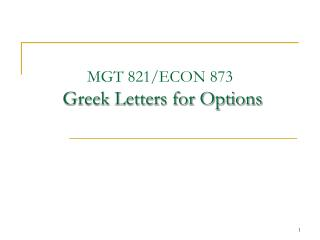# MGT 821/ECON 873 Greek Letters for Options - PowerPoint PPT PresentationDownload PresentationMGT 821/ECON 873 Greek Letters for Options

MGT 821/ECON 873 Greek Letters for OptionsDownload Presentation## MGT 821/ECON 873 Greek Letters for Options

- - - - - - - - - - - - - - - - - - - - - - - - - - - E N D - - - - - - - - - - - - - - - - - - - - - - - - - - -
##### Presentation Transcript

1. MGT 821/ECON 873 Greek Letters for Options

2. Example • A bank has sold for \$300,000 a European call option on 100,000 shares of a non-dividend paying stock • S0 = 49, K = 50, r = 5%, s = 20%, T = 20 weeks, m = 13% • The Black-Scholes value of the option is \$240,000 • How does the bank hedge its risk to lock in a \$60,000 profit?

3. Naked & Covered Positions Naked position Take no action Covered position Buy 100,000 shares today Both strategies leave the bank exposed to significant risk

4. Stop-Loss Strategy This involves: • Buying 100,000 shares as soon as price reaches \$50 • Selling 100,000 shares as soon as price falls below \$50 This deceptively simple hedging strategy does not work well

5. Stop-Loss Strategy

6. Option price Slope = D B Stock price A Delta • Delta (D) is the rate of change of the option price with respect to the underlying

7. Delta Hedging • This involves maintaining a delta neutral portfolio • The delta of a European call on a non-dividend paying stock isN (d1) • The delta of a European put on the stock is N (d1) – 1

8. Delta

9. Delta as a function of time to maturity

10. Delta Hedging … • The hedge position must be frequently rebalanced • Delta hedging a written option involves a “buy high, sell low” trading rule

11. Theta • Theta (Q) of a derivative (or portfolio of derivatives) is the rate of change of the value with respect to the passage of time • The theta of a call or put is usually negative. This means that, if time passes with the price of the underlying asset and its volatility remaining the same, the value of a long option declines

12. Theta as a function of the underlying price

13. Theta as a function of maturity

14. Gamma • Gamma (G) is the rate of change of delta (D) with respect to the price of the underlying asset • Gamma is greatest for options that are close to the money

15. Gamma Addresses Delta Hedging Errors Caused By Curvature Call price C'' C' C Stock price S S'

16. Interpretation of Gamma • For a delta neutral portfolio, DP»QDt + ½GDS2 DP DP DS DS Positive Gamma Negative Gamma

17. Relationship Between Delta, Gamma, and Theta For a portfolio of derivatives on a stock paying a continuous dividend yield at rate q

18. Vega • Vega (n) is the rate of change of the value of a derivatives portfolio with respect to volatility • Vega tends to be greatest for options that are close to the money

19. Managing Delta, Gamma, & Vega • D can be changed by taking a position in the underlying • To adjust G & n it is necessary to take a position in an option or other derivative

20. Example

21. Rho • Rho is the rate of change of the value of a derivative with respect to the interest rate • For currency options there are 2 rhos

22. Hedging in Practice • Traders usually ensure that their portfolios are delta-neutral at least once a day • Whenever the opportunity arises, they improve gamma and vega • As portfolio becomes larger hedging becomes less expensive

23. Scenario Analysis A scenario analysis involves testing the effect on the value of a portfolio of different assumptions concerning asset prices and their volatilities

24. Greek Letters for Options on an Asset that Provides a Dividend Yield at Rate q

25. Futures Contract Can Be Used for Hedging • The delta of a futures contract on an asset paying a yield at rate q is e(r-q)T times the delta of a spot contract • The position required in futures for delta hedging is therefore e-(r-q)T times the position required in the corresponding spot contract

26. Hedging vs Creation of an Option Synthetically • When we are hedging we take positions that offset D, G, n, etc. • When we create an option synthetically we take positions that match D, G, &n

27. Portfolio Insurance • In October of 1987 many portfolio managers attempted to create a put option on a portfolio synthetically • This involves initially selling enough of the portfolio (or of index futures) to match the D of the put option

28. Portfolio Insurancecontinued • As the value of the portfolio increases, the D of the put becomes less negative and some of the original portfolio is repurchased • As the value of the portfolio decreases, the D of the put becomes more negative and more of the portfolio must be sold

29. Portfolio Insurancecontinued The strategy did not work well on October 19, 1987...